# Kaggle competition report. ECML PKDD 2015 Taxi Trajectory Prediction

13 January 2017

Kaggle competitions provide a fun and useful way of exploring different datascience problems and techniques. In this blog post, I focus on one particularly interesting competition, ECML/PKDD 15: Taxi Trajectory Prediction, where the goal is to predict the destination of taxi trajectories in the city of Porto, Portugal, with maximum accuracy. The winners of that competition published an interesting summary of their solution on Kaggle's blog. They also published a detailed paper as well as their full implementation using Blocks, a framework built on top of Theano. I found their solution pretty interesting — a simple neural network combined with a clever clustering algorithm. So, just for fun, I decided to try and re-implement that solution using different frameworks, namely Keras and Tensorflow. Below I present my own implementation as well as several visualizations of the competition's dataset. As you follow along, you may also refer to my full implementation (including all the functions in the code package used in the code samples below) that I've published on Github: https://github.com/jphalip/ECML-PKDD-2015

First, let's import all the libraries we need:

In :
import pickle
import csv
import calendar
import pandas as pd
import numpy as np
import seaborn as sns
import matplotlib
import matplotlib.pyplot as plt
from matplotlib.ticker import  MultipleLocator, FormatStrFormatter
from scipy.interpolate import spline
from IPython.core.display import display_html
from code.utils import np_haversine, density_map, get_clusters, plot_embeddings
from code.training import start_new_session, process_features, create_model

# Display plots inline
%matplotlib inline

# Fix random seed for reproducibility
np.random.seed(42)


... then load the whole dataset:

In :
data = load_data()


For the sake of brevity, I won't go into all the details of how I've cleaned up and pre-processed that data in this blog post. For the full details, please refer to the full implementation of the load_data function available on Github.

The original training dataset contains nearly 1.7 million records of taxi trips. The competition's organizers provided a really tiny test dataset with only 320 records, which is too small to properly train our model. So, following the proportions described in the winners' paper, I've split the dataset into roughly 98% training, 1% validation and 1% test datasets:

In :
print data.train.shape
print data.validation.shape
print data.test.shape

(1611521, 17)
(16444, 17)
(16445, 17)


# Exploring the data¶

Now let's take a deeper look into our dataset. First, here are the available columns with all the pre-processed features:

In :
data.train.head(3)

Out:
TRIP_ID CALL_TYPE ORIGIN_CALL ORIGIN_STAND TAXI_ID TIMESTAMP POLYLINE START_LAT START_LONG QUARTER_HOUR DAY_OF_WEEK WEEK_OF_YEAR DURATION ORIGIN_CALL_ENCODED TAXI_ID_ENCODED ORIGIN_STAND_ENCODED POLYLINE_FULL
1337703 1397770496620000648 B NaN 15.0 20000648 2014-04-17 21:34:56 [(41.148531, -8.585649), (41.148594, -8.585631... 41.148531 -8.585649 86 3 15 780 0 401 15 [(41.148531, -8.585649), (41.148594, -8.585631...
1619778 1402607008620000157 B NaN 40.0 20000157 2014-06-12 21:03:28 [(41.153823, -8.67411), (41.154237, -8.673912)... 41.153823 -8.674110 84 3 23 675 0 107 40 [(41.153823, -8.67411), (41.154237, -8.673912)...
1702294 1404009216620000557 B NaN 27.0 20000557 2014-06-29 02:33:36 [(41.147703, -8.608734), (41.147757, -8.608482... 41.147703 -8.608734 10 6 25 645 0 349 27 [(41.147703, -8.608734), (41.147757, -8.608482...

One key feature is the ORIGIN_STAND column, i.e. the unique identifiers of official stands where customers may catch a taxi from. Roughly 48% of taxi rides are in fact started from official taxi stands, which is pretty significant:

In :
# Percentage of taxi rides started at taxi stands
100 * pd.notnull(data.train['ORIGIN_STAND']).sum() / float(data.train.shape)

Out:
48.00185663109572

Here is how the ORIGIN_STAND feature is distributed across the entire dataset:

In :
plt.figure(figsize=(7.5,4))
plt.xticks(rotation=90, fontsize=7)
sns.countplot(data.train['ORIGIN_STAND'].dropna().astype(int))
plt.show()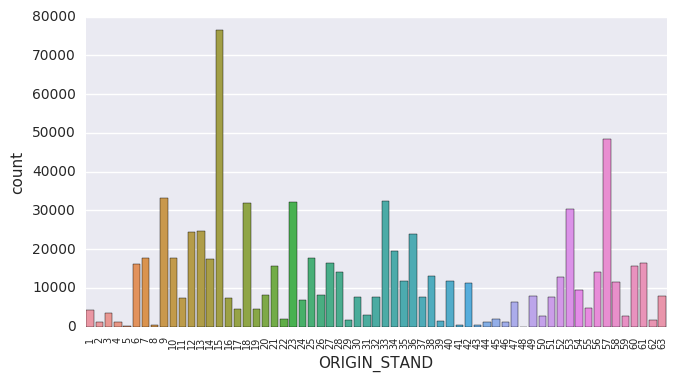Interestingly —though quite logically— the most popular taxi stands are IDs 15 and 57, i.e. Porto's two main train stations, Campanhã and São Bento, as you can see by clicking the links below:

In :
for stand_id in [15, 57]:
lat, long = data.train[data.train['ORIGIN_STAND'] == stand_id][['START_LAT', 'START_LONG']].mean()
display_html(
lat=lat, long=long, stand_id=stand_id), raw=True)


Moreover, it's probably fair to assume that people would have different ways of using taxi services depending on what period of the year, which day of the week, or what time of the day it is. Therefore I've extracted several discrete features from the provided TIMESTAMP column (i.e. the time at which each taxi ride started), respectively: week of the year (from 0 to 51), day of the week (from 0 to 6), and quarter hour of the day (from 0 to 95). Pandas makes this really easy with the DatetimeIndex:

datetime_index = pandas.DatetimeIndex(dataframe['TIMESTAMP'])
dataframe['WEEK_OF_YEAR'] = datetime_index.weekofyear - 1
dataframe['DAY_OF_WEEK'] = datetime_index.dayofweek
dataframe['QUARTER_HOUR'] = datetime_index.hour * 4 + datetime_index.minute / 15


Now let's see how all taxi trips are distributed across each week of the year:

In :
plt.figure(figsize=(7.5,4))
sns.countplot(data.train['WEEK_OF_YEAR'])
plt.gca().xaxis.set_major_locator(MultipleLocator(10))
plt.gca().xaxis.set_major_formatter(FormatStrFormatter('%d'))
plt.xlabel('Week of the year')
plt.show()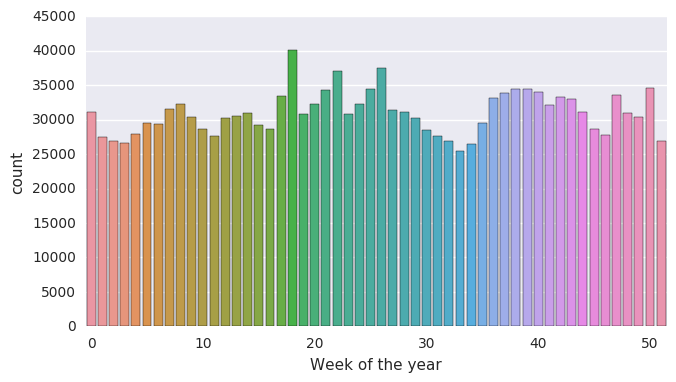The graph above has a somewhat regular wavy pattern, seeming to indicate that customers tend to use taxis more at certain periods of each month. There's also a dip around mid-August, presumably due to people leaving the city for their summer vacation.

Now looking at the distribution of taxi trips across each day of the week:

In :
plt.figure(figsize=(7.5,4))
sns.countplot(data.train['DAY_OF_WEEK'])
plt.gca().set_xticklabels(calendar.day_name)
plt.xticks(fontsize=8)
plt.xlabel('Day of the week')
plt.show()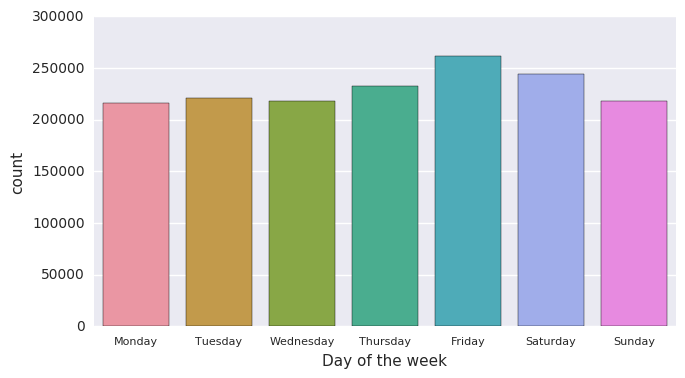... and across each quarter hour of the day:

In :
plt.figure(figsize=(7.5,4))
sns.countplot(data.train['QUARTER_HOUR'], color='royalblue')
plt.gca().xaxis.set_major_locator(MultipleLocator(10))
plt.gca().xaxis.set_major_formatter(FormatStrFormatter('%d'))
plt.xticks(fontsize=9)
plt.xlabel('Quarter hour of the day')
plt.show()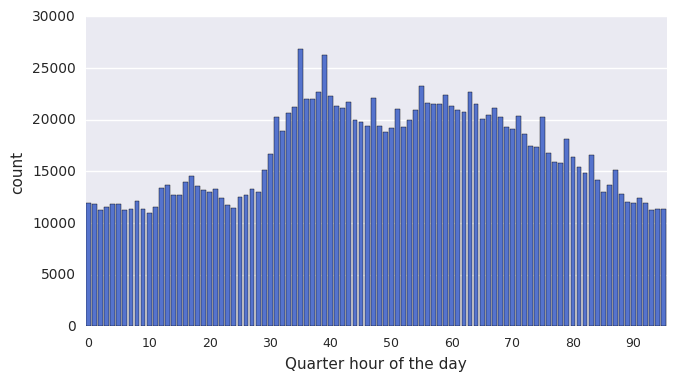From the two graphs above, we see peaks of taxi usage on Fridays and Saturdays (presumably by people going out for entertainment) and around 10am and early evening (presumably by people going to and from work). Nothing too surprising here.

Now we can take a look at the durations of trips, the majority of which are around 8 minutes:

In :
plt.figure(figsize=(7.5,4))
bins = np.arange(60, data.train.DURATION.max(), 60)
binned = pd.cut(data.train.DURATION, bins, labels=bins[:-1]/60, include_lowest=True)
sns.countplot(binned, color='royalblue')
plt.gca().xaxis.set_major_locator(MultipleLocator(5))
plt.gca().xaxis.set_major_formatter(FormatStrFormatter('%d'))
plt.xlim(-1, 40)
plt.xticks(fontsize=9)
plt.xlabel('Duration (in minutes)')
plt.show()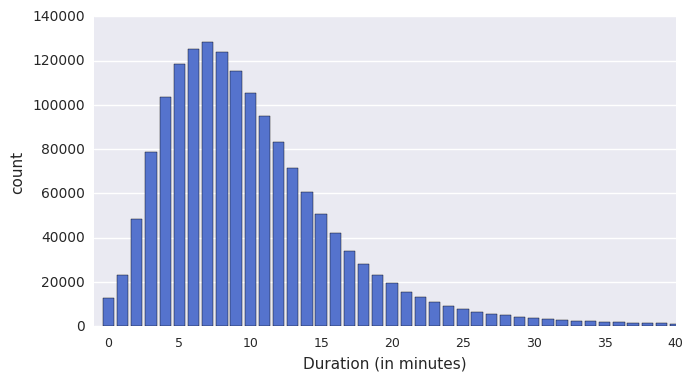Finally, it's interesting to get a visual representation of the dataset's spatial distribution (or density). This is quite simple and efficient to achieve in Python with a Matplotlib histogram. I've recently described in details how this works in an earlier blog post, so here I'll just show the result:

In :
all_coords = np.concatenate(data.train['POLYLINE_FULL'].as_matrix())
density_map(all_coords[:,0], all_coords[:,1])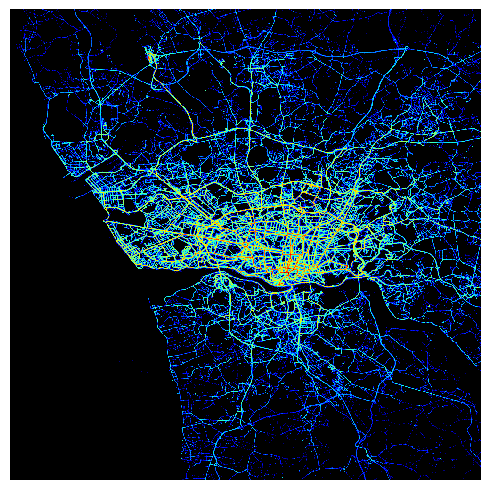This rendering process is pretty interesting as it clearly highligthts Porto's busiest roads, the airport in the North West corner, as well as the coast line and the river estuary contours.

# Building the model¶

As I mentioned in the introduction, the model that won the competition is in fact pretty simple. It is a neural network with a single hidden layer of 500 neurons and a Rectifier Linear Unit (ReLU) activation function. The input layer is comprised of embedding vectors learned for each key feature (quarter hour of the day, day of the week, week of the year, the client IDs, the taxi IDs and the stand IDs), as well as the first 5 and latest 5 recorded GPS coordinates for each taxi trip.

Where it gets particularly interesting is around the output layers. For this, the winning team's approach was threefold:

• Before training the model, do a bit of pre-processing by estimating the most popular destination points (a few thousands of them) using a mean-shift clustering algorithm.
• Use the softmax activation function as the second-to-last output layer to determine the probabilities of the destination being any of the calculated clusters.
• In the last output layer, multiply the probabilities with the clusters' coordinates to obtain the destination prediction.

The model uses a simple stochastic gradient descent optimizer. The loss function, as advised in the competition's rules, is the Haversine formula to calculate the mean distance between the predicted and actual destination points.

Here is how I've implemented this model using Keras:

def create_model(metadata, clusters):
"""
Creates all the layers for our neural network model.
"""

# Arbitrary dimension for all embeddings
embedding_dim = 10

# Quarter hour of the day embedding
embed_quarter_hour = Sequential()

# Day of the week embedding
embed_day_of_week = Sequential()

# Week of the year embedding
embed_week_of_year = Sequential()

# Client ID embedding
embed_client_ids = Sequential()

# Taxi ID embedding
embed_taxi_ids = Sequential()

# Taxi stand ID embedding
embed_stand_ids = Sequential()

# GPS coordinates (5 first lat/long and 5 latest lat/long, therefore 20 values)
coords = Sequential()

# Merge all the inputs into a single input layer
model = Sequential()
embed_quarter_hour,
embed_day_of_week,
embed_week_of_year,
embed_client_ids,
embed_taxi_ids,
embed_stand_ids,
coords
], mode='concat'))

# Simple hidden layer

# Determine cluster probabilities using softmax

# Final activation layer: calculate the destination as the weighted mean of cluster coordinates
cast_clusters = K.cast_to_floatx(clusters)
def destination(probabilities):
return tf.matmul(probabilities, cast_clusters)

# Compile the model
optimizer = SGD(lr=0.01, momentum=0.9, clipvalue=1.)  # Use clipvalue to prevent exploding gradients
model.compile(loss=tf_haversine, optimizer=optimizer)

return model


To estimate all the destination clusters efficiently, I've decided to follow two steps:

clusters = pd.DataFrame({
'approx_latitudes': destinations[:,0].round(4),
'approx_longitudes': destinations[:,1].round(4)
})

• Second, use the mean-shift algorithm available in the scikit-learn library to further reduce the number of clusters (Note: the quantile parameter was tuned to find a significant and reasonable number of clusters):
bandwidth = estimate_bandwidth(clusters, quantile=0.0002)
ms = MeanShift(bandwidth=bandwidth, bin_seeding=True)
ms.fit(clusters)
clusters = ms.cluster_centers_


Let's now see how many clusters were estimated with this approach:

In :
# Estimate clusters from all destination points
clusters = get_clusters(data.train_labels)
print("Number of estimated clusters: %d" % len(clusters))

Number of estimated clusters: 5451


We can now visualize those clusters graphically on the map:

In :
plt.figure(figsize=(6,6))
plt.scatter(clusters[:,1], clusters[:,0], c='#cccccc', s=2)
plt.axis('off')
plt.gca().xaxis.set_visible(False)
plt.gca().yaxis.set_visible(False)
plt.gca().autoscale_view('tight')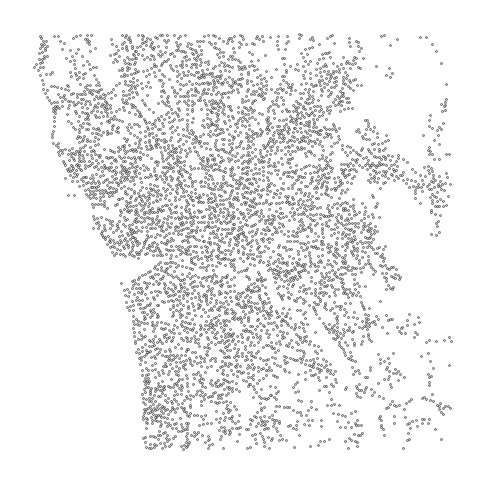Here is another visualization of those same clusters (this time in green) with all the destinations superimposed on them (in black):

In :
plt.figure(figsize=(6,6))
plt.scatter(clusters[:,1], clusters[:,0], c='#99cc99', edgecolor='None', alpha=0.7, s=40)
plt.scatter(data.train_labels[:,1], data.train_labels[:,0], c='k', alpha=0.2, s=1)
plt.grid('off')
plt.axis('off')
plt.gca().xaxis.set_visible(False)
plt.gca().yaxis.set_visible(False)
plt.gca().autoscale_view('tight')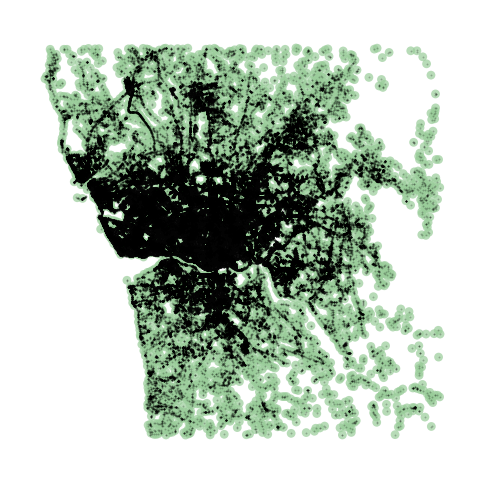# Training the model¶

For this project I've trained the model over 200 epochs. Overall, the process took about an hour an a half to run on a GeForce GTX 1070 GPU. The train and validation loss values were recorded at each epoch and saved in a pickle file for future analysis. Those values can be visualized as follows:

In :
with open('history.pickle', 'rb') as handle:

# Interpolate a smooth curve from the raw validation loss
n_epochs = len(history['val_loss'])
x_smooth = np.linspace(0, n_epochs-1, num=10)
y_smooth = spline(range(n_epochs), history['val_loss'], x_smooth)

plt.figure(figsize=(7.5,4))
plt.plot(history['loss'])
plt.plot(history['val_loss'])
plt.plot(x_smooth, y_smooth)
plt.title('Evolution of loss values during training')
plt.ylabel('loss')
plt.xlabel('epoch')
plt.xticks(fontsize=9)
plt.axes().xaxis.set_major_locator(MultipleLocator(10))
plt.legend(['train', 'validation', 'smoothened validation'], loc='upper right')
plt.show()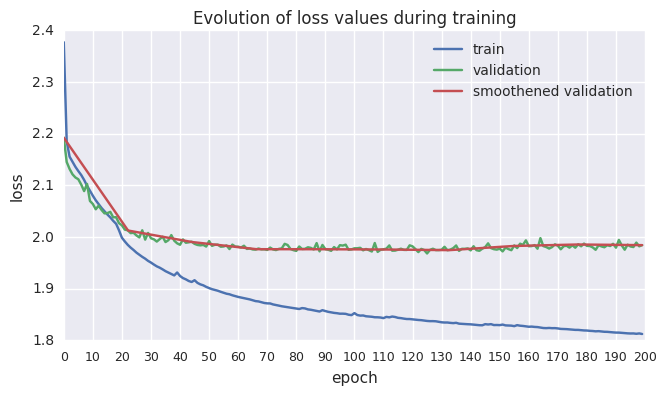The curves above show the evolution of the train and validation losses over 200 epochs. A smoothened version of the validation loss evolution is also pictured to better illustrate its trend over time.

While the train loss predictibly drops consistently and steeply, the learning process seems to reach convergence around epoch #70, at which point the validation loss stabilizes before it starts to increase. Under those circumstances, I've selected the weights learned at epoch #70 for my final model, which can now be loaded again with Keras:

In :
start_new_session()


We can now see the exact mean loss for our custom validation dataset:

In :
validation_predictions = model.predict(process_features(data.validation))
np_haversine(validation_predictions, data.validation_labels).mean()

Out:
1.9707780925774365

... and for our custom test dataset:

In :
test_predictions = model.predict(process_features(data.test))
np_haversine(test_predictions, data.test_labels).mean()

Out:
2.0029187354900984

That is roughly an average of 2km distance between the actual and predicted destination points.

# Future improvements¶

While the presented model provides encouraging results, there's clearly still room for improvement. Some avenues that could be explored include:

• Try different topologies for the neural network.
• Tweak the number of clusters.
• Incorporate ensemble models.
• Augment the training dataset by creating multiple random partial variations of the GPS trajectories.
• Engineer and include more features, for example:
• weather data (e.g. recorded temperature and rainfall)
• official holidays in Portugal
• occurrence of large events such as concerts or soccer games

I'd like to thank the competition's winning team for sharing their solution. I encourage anyone interested in spatial datasets to work on this competition as it's fun, challenging and interesting. Again, feel free to take a look at the implementation that I've published on Github — I hope that it provides some useful starting points for your own implementation strategies.